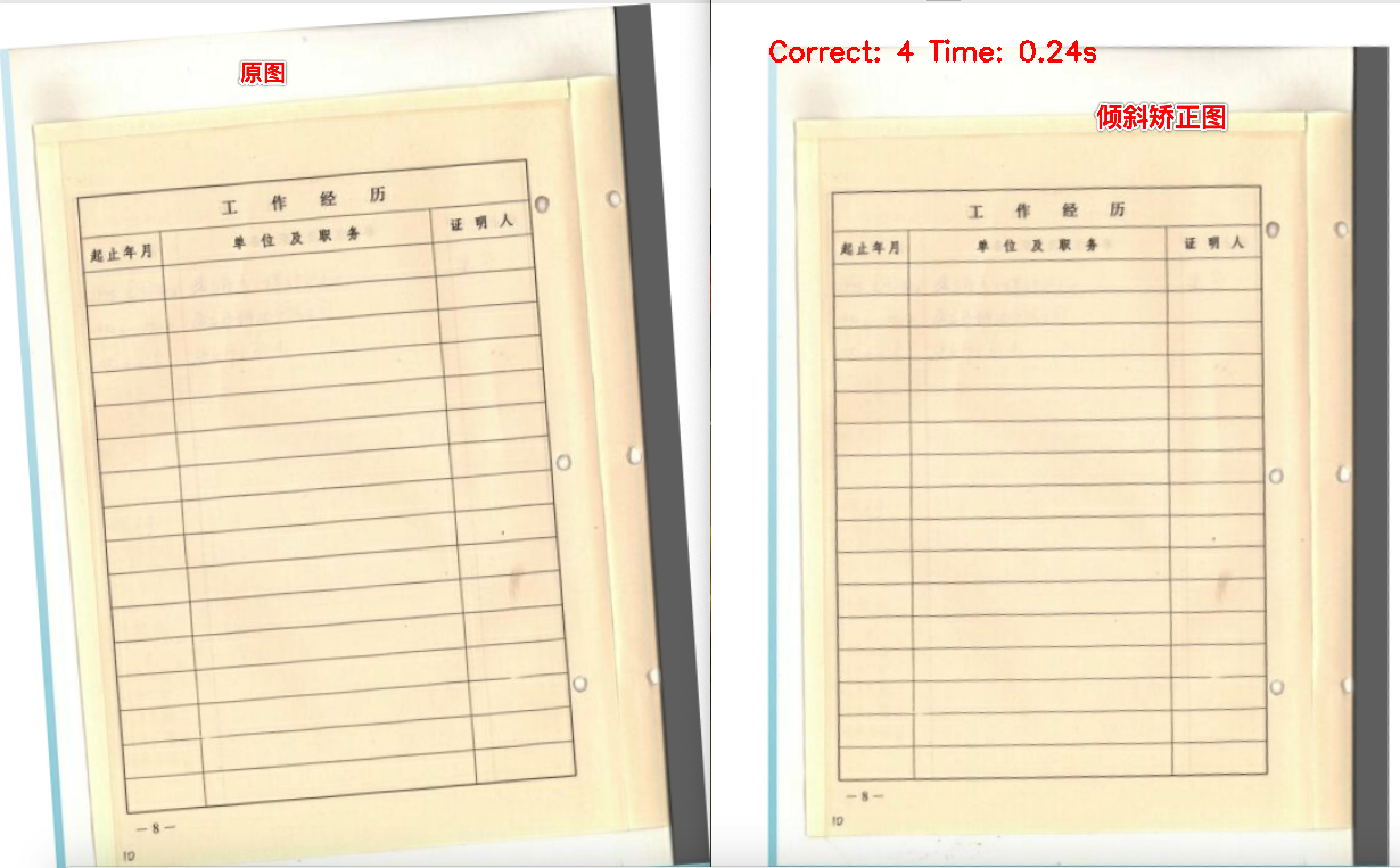2020/07/18 - 次.

# 基于Hough变换原理的文档图像倾斜角检测与校正

### 代码实现

# 像素间隔
# pixel = 3
pixel = int(image.shape * 0.0015)
if pixel == 0: pixel = 1

img_blur = cv2.cvtColor(cv2.medianBlur(image, 5), cv2.COLOR_BGR2GRAY)

# 基于双边滤波器的图像自适应阈值
# img = cv2.cvtColor(image, cv2.COLOR_BGR2GRAY)
# img = cv2.bilateralFilter(img, 9, 75, 75)

pMax = round(math.sqrt(m ** 2 + n ** 2))
thetaMax = 180
countMatrix = np.zeros((pMax, thetaMax))

for i in range(0, m, pixel):
for j in range(0, n, pixel):
for theta in range(thetaMax):
p = math.floor(abs(i * math.cos(3.14 * theta / 180) + j * math.sin(3.14 * theta / 180)))
countMatrix[p + 1, theta] = countMatrix[p + 1, theta] + 1

m, n = countMatrix.shape
angle = 0
for i in range(0, m, pixel):
for j in range(n):
if countMatrix[i, j] > countMatrix[1, 1]:
countMatrix[1, 1] = countMatrix[i, j]
angle = j•  [Richard O. Duda and Peter E. Hart. Use of the Hough Transformation to Detect Lines and Curves in Pictures (PDF). Artificial Intelligence Center (SRI International). April 1971.]
•  [荆雷，张欣，郭金鑫．基于版面的拍照文档图像倾斜校正.激光与红外[J]．2010，第10期]